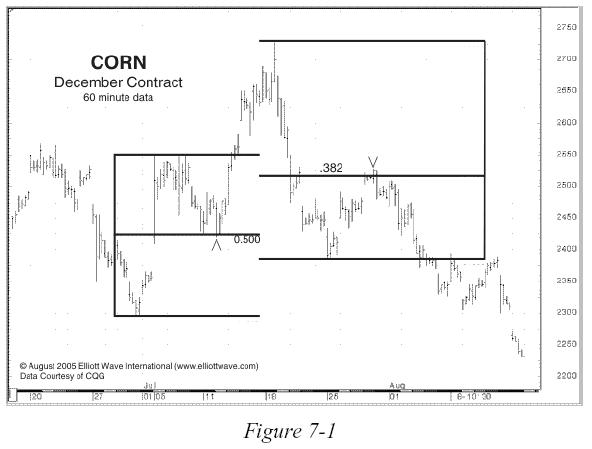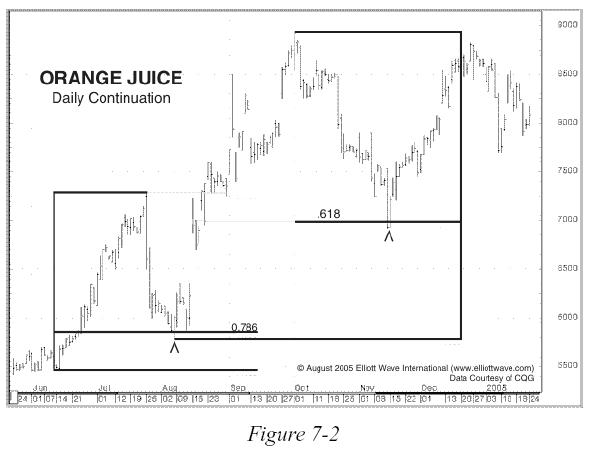# Fibonacci Techniques for Math Geeks and Everyone Else, Too

By Editorial Staff

The word Fibonacci (pronounced fib-oh-notch-ee) can draw either blank stares or an enthusiastic response. There's hardly any in-between ground. But for those who ask how an esoteric mathematical relationship can apply to price charts and trading, here's a quick lesson. Everyone who uses Elliott wave analysis will sooner or later want to try using Fibo techniques, and Elliott Wave International's Jeff Kennedy has written about five of them in a Trader's Classroom column. For an example of why people are so fascinated by Fibonacci, read part of Kennedy's article here:

* * * * *

How to Apply Fibonacci Math to Real-World Trading
Have you ever given an expensive toy to a small child and watched while the child had less fun playing with the toy than with the box that it came in? In fact, I can remember some of the boxes I played with as a child that became spaceships, time machines or vehicles to use on dinosaur safaris.

In many ways, Fibonacci math is just like the box kids enjoy playing with imaginatively for hours on end. It's hard to imagine a wrong way to apply Fibonacci ratios or multiples to financial markets, and new ways are being tested every day. Let's look at just some of the ways I apply Fibonacci math in my own analysis.

Fibonacci Retracements
Financial markets demonstrate an uncanny propensity to reverse at certain Fibonacci levels. The most common Fibonacci ratios I use to forecast retracements are .382, .500 and .618. On occasion, I find .236 and .786 useful, but I prefer to stick with the big three. You can imagine how helpful these can
be: Knowing where a corrective move is likely to end often identifies high-probability trade setups (Figures 7-1 and 7-2).Kennedy then goes on to explain Fibonacci extensions, circles, fans and time, using 11 charts to show what he means. Whether or not you are a math geek, you can learn a lot from this six-page introduction to Fibonacci math.

Get Your Fibonacci Techniques Right Here
. Jeffrey Kennedy has been using and teaching these techniques for years, and he has written a quick description of five Fibonacci techniques in his Trader's Classroom column — now available to you for free by signing up as a Club EWI member. Read more